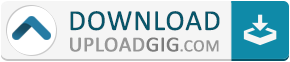#Do not remember me!

### Categories## TechCalc+ Scientific Calculator (adfree) v4.4.9Requirements: Android 4.0+ | Size: 12,1 MB

TechCalc+ contains 12 computing modes in one application + a handy reference section.

Modes included are:
* Basic Mode,
* Scientific Mode,
* 64-bit Programmer Mode (Hex, Oct, Bin and Dec),
* Graphing (save copy of graph to sdcard),
* Matrices,
* Complex Numbers (cartesian, polar, using Euler's identity),
* Quick Formulas,
* Quick Converter,
* Time Calculator,
* Equation Solver,
* Calculus (Derivative, Definite Integral, Taylor Series & Indefinite Integral) - requires Android version 2.3 or higher
* Financial

+ the Periodic Table of Elements!

Features include:
* Powers & Roots
* Logs and Antilogs
* Factorial, Modulus & Random Numbers functions
* HCF, LCM, Prime factorization
* Pol() & Rec() Functions
* Permutations (nPr) and Combinations (nCr)
* Statistics
* Fractions Mode
* A wide range of conversion categories and constants
* 20 Memory Registers in each of the calculation modes
* Detailed calculation history
* Extensive Help and Reference
* Highly customizable via the Settings

The reference section includes:
* Physical Laws
* Names in the Metric System
* Mathematical Tables
* Elementary & Linear Algebra
* Trigonometric Identities
* Differentiation & Integration Rules
* Statistics Formulas
* Vector Mathematics
* ASCII, Fractional Bits, Roman Numeral & Number Base Converters
* pH, Interpolation, Body Mass Index (BMI), Percentage, Proportion & Molecular Weight Calculators
* Sigma & Pi Notation
* Balancing Chemical Equations
* Statistics (Grouped Data)
* Numerical Sequences
* Humidity Calculations
* Boolean Algebra Calculator
* Empirical Formula Calculator
* Characteristics of an RLC Circuit
* Feet and Inches CalculatorWHAT'S NEW:
4.4.9:
* added Coordinates Converter and Unit Price Comparison to the Reference Section
* added additional means of stack manipulation in RPN mode - please see the topic "Reverse Polish Notation (RPN)" in the Help Section for details
* added ability to edit, and display a running total for, simple calculations on the main screen of Financial modes

Homepage